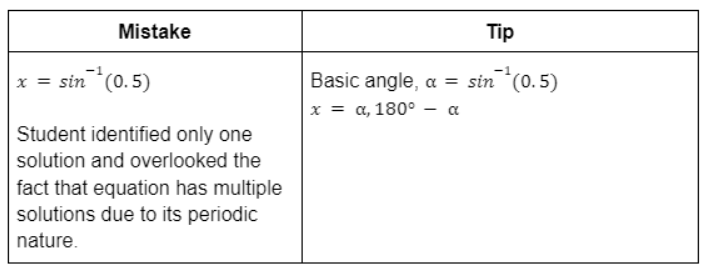top of page
Search

# 5 Common O Level Maths Trigonometry Mistakes and How to Avoid ThemTrigonometry is a fundamental branch of Mathematics that often challenges O Level students. As parents, it’s essential to understand the common pitfalls students might encounter when working on O Level Maths trigonometry questions. In this article, we’ll delve into five common mistakes that students often make and provide helpful tips to assist your child

in overcoming these challenges.

## 1. Misinterpreting Inverse Trigonometric Functions:

Inverse trigonometric functions, such as tan-1, sin-1, and cos-1, play a vital role in solving trigonometric equations. However, students might misinterpret their use, leading to incorrect solutions. For example, consider the equation tan-1(x) = 0.5. Students might mistakenly treat tan-1(x) as 1/tan(x), leading to a wrong interpretation. To avoid this mistake, remind your child that tan-1(x) is an inverse trigonometric function and should be treated as such. The correct interpretation of the example equation is x = tan(0.5).

Example: Solve for x in the equation tan-1(x)=0.5.## 2. Misinterpreting Powers of Trigonometric Functions

Another common mistake arises from misinterpreting powers of trigonometric functions. For instance, if your child encounters the equation sin x2 = 0.5, they might incorrectly treat sin x2 as (sin x)2. To steer clear of this error, encourage your child to recognise that sin x2 is not the same as sin2 x. In this scenario, the correct approach is to solve for sin x2 directly, as shown in the example sin x2 = 0.5.

Example: Solve for x in the equation sin x2=0.5.Quadrant considerations are crucial in trigonometry, especially when dealing with inverse trigonometric functions. Students may overlook this aspect, resulting in incomplete solutions. Imagine the equation sin⁡(x) = 0.5, where 0° ≤ x ≤ 360°. If your child only identifies one solution, they might miss the fact that trigonometric equations often have multiple solutions due to the periodic nature of trigonometric functions. To avoid this error, teach your child to consider the entire range of angles within the given constraints, as demonstrated in the example.

Example: Solve for x in the equation sin x=0.5, where 0°≤x≤360°## 4. Ignoring the Given Range of Angles

Sometimes, students neglect the range of angles given in the question, leading to solutions that are incomplete or incorrect. For instance, when solving sin⁡(2x) = 0.5 with 0° ≤ x ≤ 360°, students might solve for angles in quadrants 1 and 2, assuming they’ve covered the entire range. However, they might overlook angles in other quadrants, resulting in an incomplete solution. To help your child avoid this error, ensure they understand the range of angles specified and apply the correct calculations accordingly, as illustrated in the example.

Example: Solve for x in the equation sin 2x=0.5, where 0°≤x≤360°## 5. Forgetting to Check the Validity of Solutions

It’s common for students to forget to check the validity of solutions, especially when dealing with complex equations involving multiple terms. In equations like (2sin x-1)(sin x+2)=0, students might fail to realise that certain values make the equation invalid due to the limited range of the sine rule function. To prevent this oversight, encourage your child to double-check their solutions against the possible range of the involved trigonometric functions. This practice ensures that the solutions are mathematically sound, as highlighted in the example.

Example: Solve for x in the equation (2sin x-1)(sin x+2)=0, where 0°≤x≤360°.By understanding and addressing these common mistakes in O Level trigonometry, you can provide valuable guidance to your child as they navigate through their Mathematical studies. Keep in mind that trigonometry is a skill that improves with practice and a deep understanding of its principles.

Encourage your child to approach each problem with patience, pay attention to the specific context of the question, and double-check their solutions for accuracy and validity. By helping them recognise and rectify these common errors, you’ll contribute to their success in Trigonometry O Level and lay a strong foundation for their future mathematical endeavours.Seeking excellence in O Level Math for your child? Choose AGrader Learning Centre! Our High-Quality Mathematics Curriculum uses a Step-by-Step Approach to tackle challenging math problems efficiently. With our unique methods, your child can solve 90% of mind-boggling math problems quickly.

AGrader Secondary Math tutors employ heuristics to develop problem-solving skills, empowering students to conquer the toughest problem sums with ease. Trigonometry, a crucial O Level topic, can be daunting – but not with AGrader. We address the 5 common trigonometry Mistakes, providing solutions for your child’s success.

Equip your child with invaluable skills, a deep grasp of core concepts, and the ability to excel in O Level Math by enrolling at AGrader’s Secondary Math Tuition Programme now!